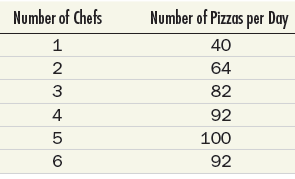# The following table shows the number of pizzas that can be produced by a large pizza parlor employing various numbers of pizza chefs. Find the marginal physical product schedule of the pizza chefs. Assuming a price of \$9 per pizza, find the marginal revenue product schedule. If chefs are paid \$100 per day, how many chefs will this pizza parlor employ? How would your answer change if chefs’ wages rose to \$125 per day? Suppose the price of pizza increases from \$9 to \$12. Show what happens to the derived demand curve for chefs.### Microeconomics: Principles & Policy

14th Edition
William J. Baumol + 2 others
Publisher: Cengage Learning
ISBN: 9781337794992

#### Solutions

Chapter
Section### Microeconomics: Principles & Policy

14th Edition
William J. Baumol + 2 others
Publisher: Cengage Learning
ISBN: 9781337794992
Chapter 19, Problem 1TY
Textbook Problem
1 views

## The following table shows the number of pizzas that can be produced by a large pizza parlor employing various numbers of pizza chefs.Find the marginal physical product schedule of the pizza chefs. Assuming a price of \$9 per pizza, find the marginal revenue product schedule. If chefs are paid \$100 per day, how many chefs will this pizza parlor employ? How would your answer change if chefs’ wages rose to \$125 per day? Suppose the price of pizza increases from \$9 to \$12. Show what happens to the derived demand curve for chefs.

This textbook solution is under construction.

### Still sussing out bartleby?

Check out a sample textbook solution.

See a sample solution

#### The Solution to Your Study Problems

Bartleby provides explanations to thousands of textbook problems written by our experts, many with advanced degrees!

Get Started